# Title 12

## Appendix J to Part 226 - Annual Percentage Rate Computations for Closed-End Credit Transactions

### 12:3.0.1.1.7.9.8.1.25 : Appendix J

Appendix J to Part 226 - Annual Percentage Rate Computations for Closed-End Credit Transactions (a) Introduction

(1) Section 226.22(a) of Regulation Z provides that the annual percentage rate for other than open end credit transactions shall be determined in accordance with either the actuarial method or the United States Rule method. This appendix contains an explanation of the actuarial method as well as equations, instructions and examples of how this method applies to single advance and multiple advance transactions.

(2) Under the actuarial method, at the end of each unit-period (or fractional unit-period) the unpaid balance of the amount financed is increased by the finance charge earned during that period and is decreased by the total payment (if any) made at the end of that period. The determination of unit-periods and fractional unit-periods shall be consistent with the definitions and rules in paragraphs (b) (3), (4) and (5) of this section and the general equation in paragraph (b)(8) of this section.

(3) In contrast, under the United States Rule method, at the end of each payment period, the unpaid balance of the amount financed is increased by the finance charge earned during that payment period and is decreased by the payment made at the end of that payment period. If the payment is less than the finance charge earned, the adjustment of the unpaid balance of the amount financed is postponed until the end of the next payment period. If at that time the sum of the two payments is still less than the total earned finance charge for the two payment periods, the adjustment of the unpaid balance of the amount financed is postponed still another payment period, and so forth.

(b) Instructions and Equations for the Actuarial Method (1) General Rule

The annual percentage rate shall be the nominal annual percentage rate determined by multiplying the unit-period rate by the number of unit-periods in a year.

(2) Term of the Transaction

The term of the transaction begins on the date of its consummation, except that if the finance charge or any portion of it is earned beginning on a later date, the term begins on the later date. The term ends on the date the last payment is due, except that if an advance is scheduled after that date, the term ends on the later date. For computation purposes, the length of the term shall be equal to the time interval between any point in time on the beginning date to the same point in time on the ending date.

(3) Definitions of Time Intervals

(i) A period is the interval of time between advances or between payments and includes the interval of time between the date the finance charge begins to be earned and the date of the first advance thereafter or the date of the first payment thereafter, as applicable.

(ii) A common period is any period that occurs more than once in a transaction.

(iii) A standard interval of time is a day, week, semimonth, month, or a multiple of a week or a month up to, but not exceeding, 1 year.

(iv) All months shall be considered equal. Full months shall be measured from any point in time on a given date of a given month to the same point in time on the same date of another month. If a series of payments (or advances) is scheduled for the last day of each month, months shall be measured from the last day of the given month to the last day of another month. If payments (or advances) are scheduled for the 29th or 30th of each month, the last day of February shall be used when applicable.

(4) Unit-period

(i) In all transactions other than a single advance, single payment transaction, the unit-period shall be that common period, not to exceed 1 year, that occurs most frequently in the transaction, except that

(A) If 2 or more common periods occur with equal frequency, the smaller of such common periods shall be the unit-period; or

(B) If there is no common period in the transaction, the unit-period shall be that period which is the average of all periods rounded to the nearest whole standard interval of time. If the average is equally near 2 standard intervals of time, the lower shall be the unit-period.

(ii) In a single advance, single payment transaction, the unit-period shall be the term of the transaction, but shall not exceed 1 year.

(5) Number of Unit-periods Between 2 Given Dates

(i) The number of days between 2 dates shall be the number of 24-hour intervals between any point in time on the first date to the same point in time on the second date.

(ii) If the unit-period is a month, the number of full unit-periods between 2 dates shall be the number of months measured back from the later date. The remaining fraction of a unit-period shall be the number of days measured forward from the earlier date to the beginning of the first full unit-period, divided by 30. If the unit-period is a month, there are 12 unit-periods per year.

(iii) If the unit-period is a semimonth or a multiple of a month not exceeding 11 months, the number of days between 2 dates shall be 30 times the number of full months measured back from the later date, plus the number of remaining days. The number of full unit-periods and the remaining fraction of a unit-period shall be determined by dividing such number of days by 15 in the case of a semimonthly unit-period or by the appropriate multiple of 30 in the case of a multimonthly unit-period. If the unit-period is a semimonth, the number of unit-periods per year shall be 24. If the number of unit-periods is a multiple of a month, the number of unit-periods per year shall be 12 divided by the number of months per unit-period.

(iv) If the unit-period is a day, a week, or a multiple of a week, the number of full unit-periods and the remaining fractions of a unit-period shall be determined by dividing the number of days between the 2 given dates by the number of days per unit-period. If the unit-period is a day, the number of unit-periods per year shall be 365. If the unit-period is a week or a multiple of a week, the number of unit-periods per year shall be 52 divided by the number of weeks per unit-period.

(v) If the unit-period is a year, the number of full unit-periods between 2 dates shall be the number of full years (each equal to 12 months) measured back from the later date. The remaining fraction of a unit-period shall be

(A) The remaining number of months divided by 12 if the remaining interval is equal to a whole number of months, or

(B) The remaining number of days divided by 365 if the remaining interval is not equal to a whole number of months.

(vi) In a single advance, single payment transaction in which the term is less than a year and is equal to a whole number of months, the number of unit-periods in the term shall be 1, and the number of unit-periods per year shall be 12 divided by the number of months in the term or 365 divided by the number of days in the term.

(vii) In a single advance, single payment transaction in which the term is less than a year and is not equal to a whole number of months, the number of unit-periods in the term shall be 1, and the number of unit-periods per year shall be 365 divided by the number of days in the term.

(6) Percentage Rate for a Fraction of a Unit-period

The percentage rate of finance charge for a fraction (less than 1) of a unit-period shall be equal to such fraction multiplied by the percentage rate of finance charge per unit-period.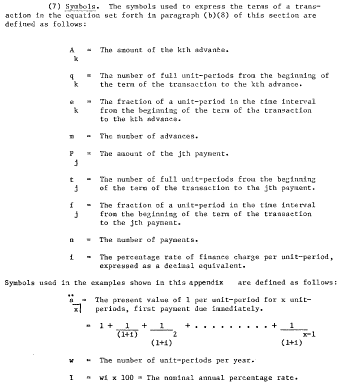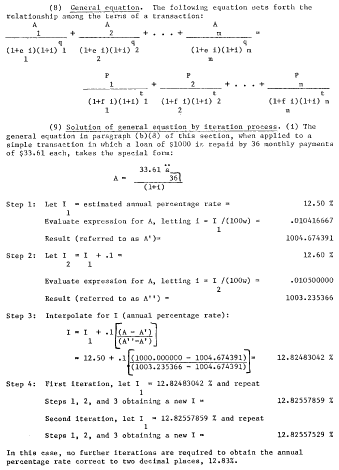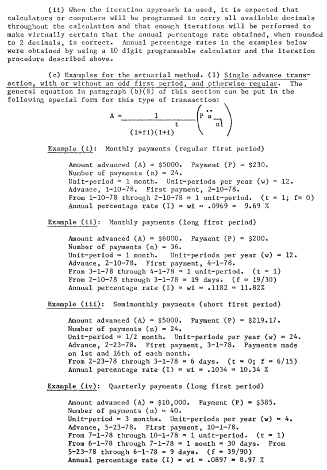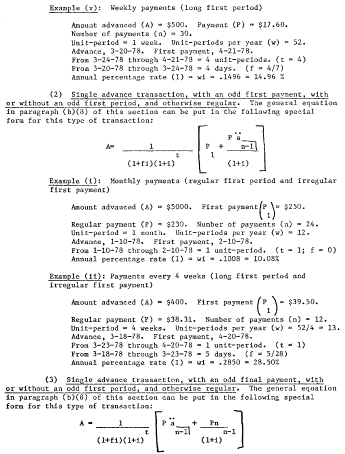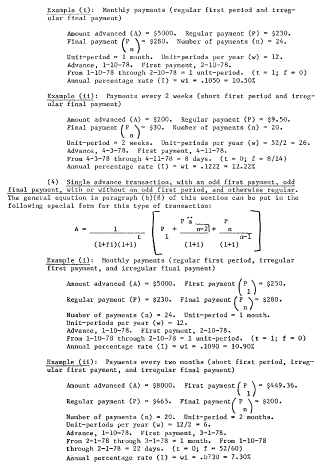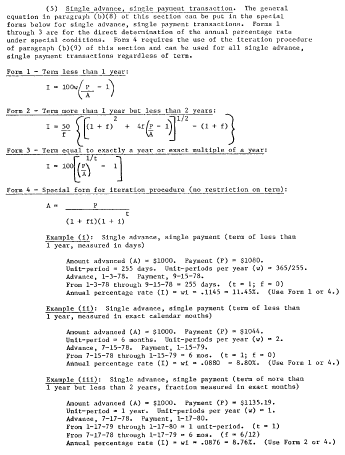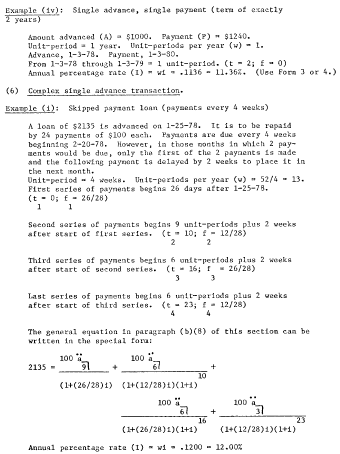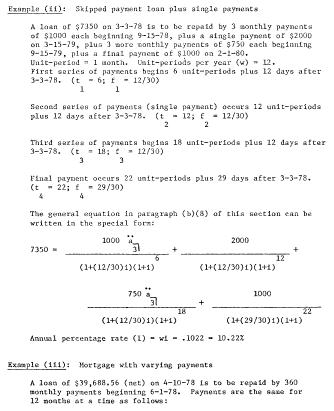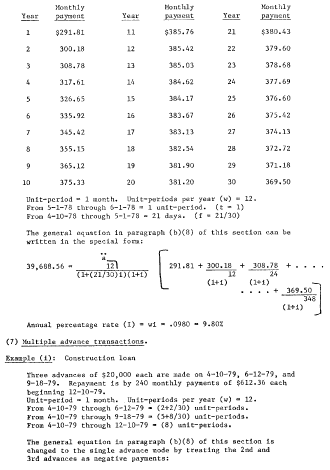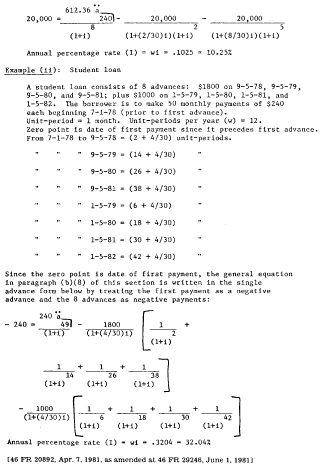[Reg. Z, 46 FR 20892, Apr. 7, 1981, as amended at 46 FR 29246, June 1, 1981]